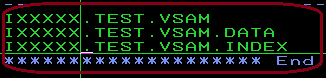## Wednesday, August 6, 2014

### Can we create a VSAM file with IEFBR14 ?

The Answer is 'Yes', we can, but with some limitations. We all know or used to IDCAMS  when it comes to VSAM creation. But even IEFBR14 can create VSAM with some advantages and disadvantages!

Let have the JCL for our first run:
//STEP1    EXEC PGM=IEFBR14
//SYSPRINT DD SYSOUT=*
//DD1      DD DISP=(,CATLG),DSN=IXXXX.TEST.VSAM
//         LRECL=80,KEYLEN=5,KEYOFF=0,RECORG=KS,
//         SPACE=(CYL,(5,5)

/*
And here you go with the Output for KSDS VSAM:Lets look for the parameters used in the JCL
LRECL=80  Defines the maximum record length of the VSAM in bytes
RECORG  Is the important parameter to note. Its value determines the type of VSAM dataset the job will create.
Here in the example we have
RECORG=KS which made it as a KSDS
RECORG=ES  will create a ESDS
RECORG=ES  will create Linear data set
RECORG=RR will create RRDS
'KEYLEN=' will define the length of the KSDS key
'KEYOFF='  will define the key offset length. Here the value of '0' indicates the first byte.
'SPACE='  will allocate the space .Here it is in cylinders, the primary and secondary being 5 each.
However we can also use SPACE=(TRK,(50,20))
We can use the AVGREC parameter along with the SPACE parameter like below.The value of AVGREC has some significance.
AVGREC=U  implies primary and secondary space numbers are  number of records
AVGREC=K  implies primary and secondary space numbers are  number of records in thousands(multiplied by1024)
AVGREC=M  implies primary and secondary space numbers are  number of records in Million(multiplied by 1024*1024)
Re writing the Above JCL once more
//STEP1    EXEC PGM=IEFBR14
//SYSPRINT DD SYSOUT=*
//DD1      DD DISP=(,CATLG),DSN=I15122.TEST.VSAM
//         LRECL=80,KEYLEN=5,KEYOFF=0,RECORG=KS,
//         SPACE=(60,(300,200)),AVGREC=U

/*
So here, the primary space large enough for  300*60=18000 bytes and secondary for 1200 bytes.